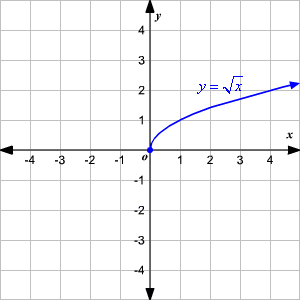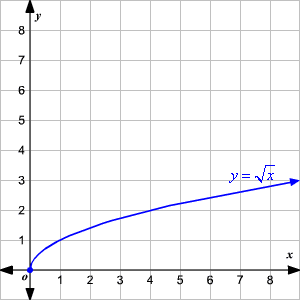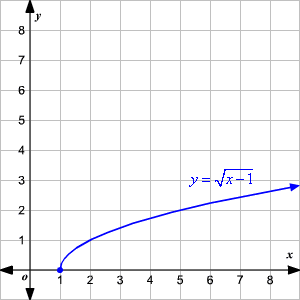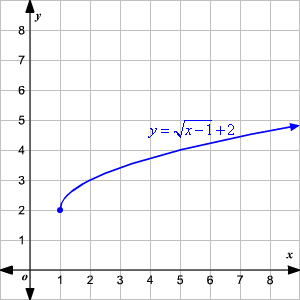# Graphing Square Root Functions

The parent function of the functions of the form $f\left(x\right)=\sqrt{x-a}+b$ is $f\left(x\right)=\sqrt{x}$ .Note that the domain of $f\left(x\right)=\sqrt{x}$ is $x\ge 0$ and the range is $y\ge 0$ .

The graph of $f\left(x\right)=\sqrt{x-a}+b$ can be obtained by translating the graph of $f\left(x\right)=\sqrt{x}$ to $a$ units to the right and then $b$ units up.

Example:

Sketch the graph of $y=\sqrt{x-1}+2$ from its parent graph $y=\sqrt{x}$ .

Solution:

Step 1: Draw the graph of $y=\sqrt{x}$ .Step 2: Move the graph of $y=\sqrt{x}$ by $1$ unit to the right to obtain the graph of $y=\sqrt{x-1}$ .Step 3: Move the graph of $y=\sqrt{x-1}$ by $2$ units up to obtain the graph of $y=\sqrt{x-1}+2$ .The domain of the function $y=\sqrt{x-1}+2$ is $x\ge 1$ .

The range of the function $y=\sqrt{x-1}+2$ is $y\ge 2$ .